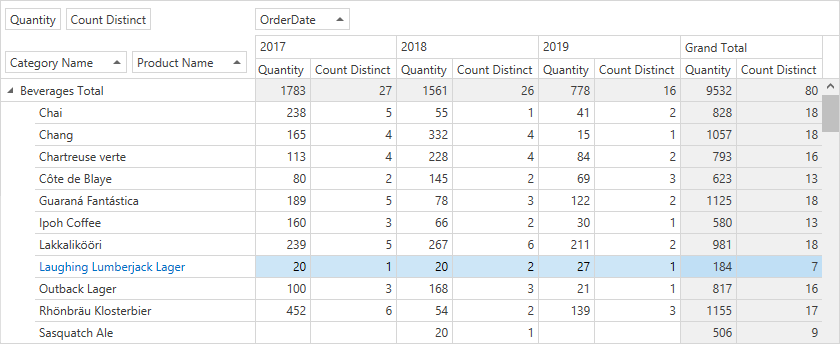# Custom Summaries

This article describes how to create custom summaries in Optimized and Server modes.

Use the PivotGridControl.CustomSummary event to create custom summaries in Legacy or LegacyOptimized mode.

## Custom Summaries Overview

Pivot Grid allows you to calculate common summary functions for data fields. You can create custom summaries to execute complex calculations.

Custom summaries can do the following:

• Use predefined and custom aggregate functions to calculate summary values.
• Include multiple fields in a summary calculation.
• Calculate a summary for records that match certain criteria.

## Create a Custom Summary

Do the following to create a custom summary for a data field:

1. Create a Pivot Grid field.
2. Create an ExpressionDataBinding object, and specify a calculated summary expression in the object’s constructor.
3. Assign the created `ExpressionDataBinding` object to the field’s DataBinding property.
4. Add the field to the Data Area.

Refer to the following article for information on how to create calculated expressions: Bind Pivot Grid Fields to Calculated Expressions.

Tip

If a custom summary calculation requires data from multiple data sources, bind the Pivot Grid to a FederationDataSource that combines data from two or more tables into one data set. Then, the custom summary expression can use fields defined in the `FederationDataSource`.

### Example

The example below shows how create a `Count Distinct` field and bind it to an expression that contains a custom function. The expression counts distinct values (the number of orders with equal product quantities).``````<dx:ThemedWindow x:Class="DXPivotGrid_CustomSummary.MainWindow" mc:Ignorable="d" Title="MainWindow" Height="450" Width="800"
xmlns:dx="http://schemas.devexpress.com/winfx/2008/xaml/core"
xmlns="http://schemas.microsoft.com/winfx/2006/xaml/presentation"
xmlns:x="http://schemas.microsoft.com/winfx/2006/xaml"
xmlns:d="http://schemas.microsoft.com/expression/blend/2008"
xmlns:mc="http://schemas.openxmlformats.org/markup-compatibility/2006"
xmlns:local="clr-namespace:DXPivotGrid_CustomSummary"
xmlns:dxpg="http://schemas.devexpress.com/winfx/2008/xaml/pivotgrid">
<Grid>

DataProcessingEngine="Optimized">
<dxpg:PivotGridControl.Fields>
<!-- ... -->
<dxpg:PivotGridField Name="fieldQuantityDistinctCount"  Area="DataArea" AreaIndex="1"
Caption="Count Distinct">
<dxpg:PivotGridField.DataBinding>
<dxpg:ExpressionDataBinding Expression="DistinctCount([OrderID])"/>
</dxpg:PivotGridField.DataBinding>
</dxpg:PivotGridField>
</dxpg:PivotGridControl.Fields>
</dxpg:PivotGridControl>

</Grid>
</dx:ThemedWindow>
``````